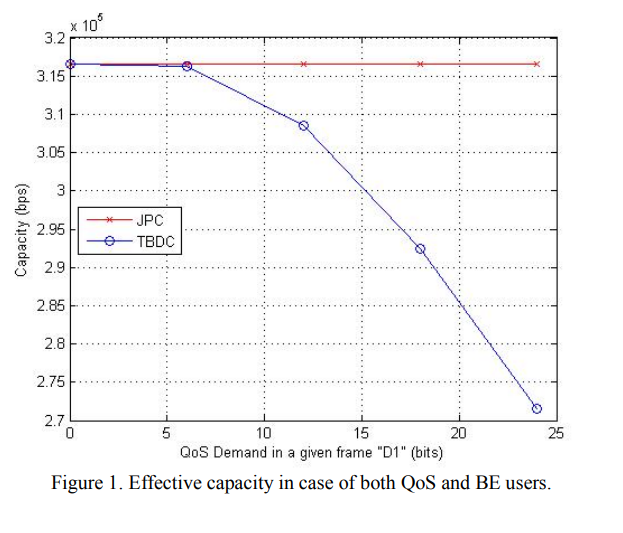Conference Paper

# Time-based demand-constrained cross-layer resource allocation for wireless networks

By
Morsy K.E.
Nafie M.H.
Digham F.F.
Elezabi A.Y.

Efficient resource allocation is a critical component in multi-user QoS communications and high speed networks. In this paper, we devise a new mathematical model for the resource allocation problem that takes into account the users' demands in a PHY-MAC cross-layer approach. Incorporating the time axis in our model, the target is to maximize the number of bits transmitted in a given frame rather than maximizing the channel capacity or the average throughput. Our design is governed by constraints on users' demands (expressed in bits), energy expenditure, and frame duration. We model the allocation problem as an optimization problem whose solution allocates channels, time span, and power levels to each user. We also derive an upper bound on the performance of any resource allocation algorithm and use this bound to assess the performance of the solution obtained using our proposed model. ©2010 IEEE.